## Exercise 11: Introduction to estimating diversification rates

In this tutorial, we will use phytools to create lineage-through-time (LTT) plots and compute the γ test statistic of Pybus & Harvey (2010).

### Lineage through time plots

To get started, let's load phytools & dependencies:

``````library(phytools)
``````

Next, let's load a tree from file: etheostoma_percina_chrono.tre.

``````darter.tree<-read.tree("etheostoma_percina_chrono.tre")
``````

Our tree is from Near et al. (2011). In it, they sampled 201 of 216 species from the group (93%) - but for the purposes of this exercise (and the next), we will assume 100% sampling. We can relax this assumption later.

Let's first plot the tree:

``````plotTree(darter.tree,ftype="i",fsize=0.4,type="fan",lwd=1)
``````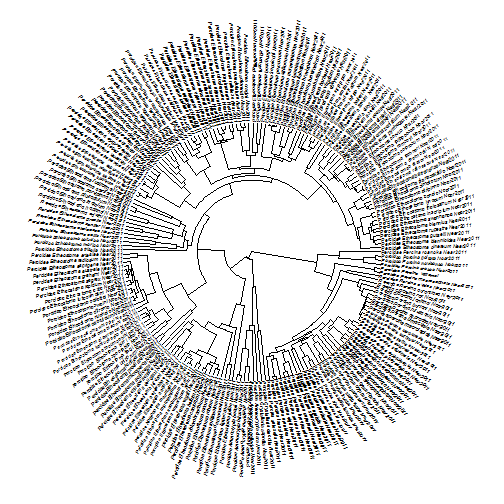Now let's use the function `ltt` in the phytools package to generate a LTT plot:

``````obj<-ltt(darter.tree,log.lineages=FALSE)
``````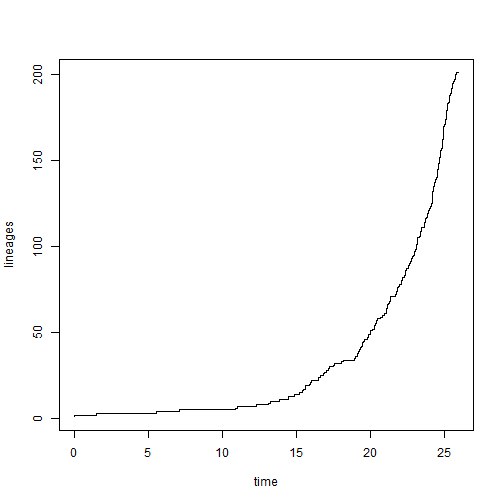``````obj
``````
``````## Object of class "ltt" containing:
##
## (1) A phylogenetic tree with 201 tips and 198 internal nodes.
##
## (2) Vectors containing the number of lineages (ltt) and branching times (times) on the tree.
##
## (3) A value for Pybus & Harvey's "gamma" statistic of NA, p-value = NA.
``````

This is weird. Well, it turns out that our tree is not fully bifurcating, so we need to resolve polytomies to continue:

``````darter.tree
``````
``````##
## Phylogenetic tree with 201 tips and 198 internal nodes.
##
## Tip labels:
##  Percidae_Etheostoma_cinereum_Near2011, Percidae_Etheostoma_blennioides_Near2011, Percidae_Etheostoma_gutselli_Near2011, Percidae_Etheostoma_rupestre_Near2011, Percidae_Etheostoma_blennius_Near2011, Percidae_Etheostoma_swannanoa_Near2011, ...
##
## Rooted; includes branch lengths.
``````
``````darter.tree<-multi2di(darter.tree)
darter.tree
``````
``````##
## Phylogenetic tree with 201 tips and 200 internal nodes.
##
## Tip labels:
##  Percidae_Etheostoma_cinereum_Near2011, Percidae_Etheostoma_blennioides_Near2011, Percidae_Etheostoma_gutselli_Near2011, Percidae_Etheostoma_rupestre_Near2011, Percidae_Etheostoma_blennius_Near2011, Percidae_Etheostoma_swannanoa_Near2011, ...
##
## Rooted; includes branch lengths.
``````
``````obj<-ltt(darter.tree,plot=FALSE)
obj
``````
``````## Object of class "ltt" containing:
##
## (1) A phylogenetic tree with 201 tips and 200 internal nodes.
##
## (2) Vectors containing the number of lineages (ltt) and branching times (times) on the tree.
##
## (3) A value for Pybus & Harvey's "gamma" statistic of 0.2007, p-value = 0.841.
``````
``````plot(obj,log.lineages=FALSE,main="LTT plot for darters")
``````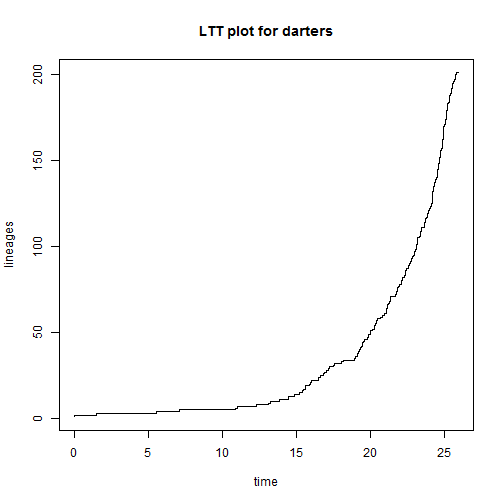It is even possible to visualize the LTT plot with the tree overlain:

``````plot(obj,show.tree=TRUE,log.lineages=FALSE,main="LTT plot for darters")
``````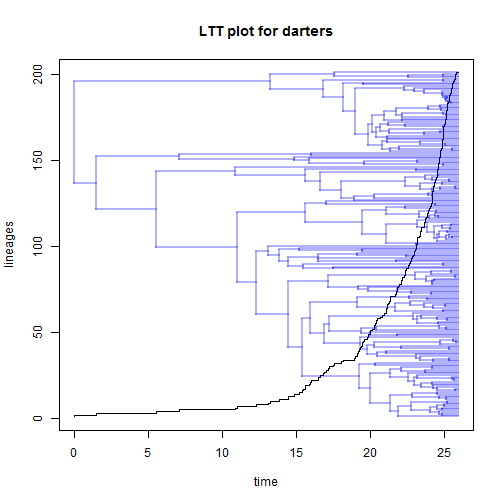Note that under pure-birth, the number of lineages should rise exponentially through time. By convention, and because it linearizes the expectation under pure-birth, we normally would plot our pure-birth trees on a semi-log scale, meaning that the vertical axis is on a log-scale while our horizontal axis is on a linear scale.

``````plot(obj,log.lineages=FALSE,log="y",main="LTT plot for darters",
ylim=c(2,Ntip(darter.tree)))
## we can overlay the pure-birth prediction:
h<-max(nodeHeights(darter.tree))
x<-seq(0,h,by=h/100)
b<-(log(Ntip(darter.tree))-log(2))/h
lines(x,2*exp(b*x),col="red",lty="dashed",lwd=2)
``````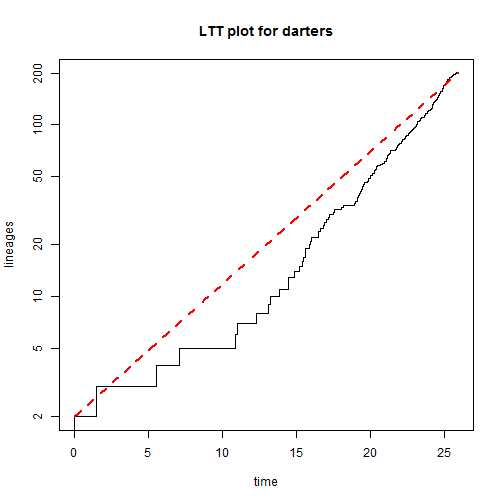We might like to compare the observed LTT, to simulated LTTs assuming a pure-birth process of the same duration & resulting in the same total number of species. We can do this using the phytools function `pbtree`:

``````trees<-pbtree(b=b,n=Ntip(darter.tree),t=h,nsim=100,method="direct",
quiet=TRUE)
obj<-ltt(trees,plot=FALSE)
plot(obj,col="grey",main="LTT of darters compared to simulated LTTs")
lines(c(0,h),log(c(2,Ntip(darter.tree))),lty="dashed",lwd=2,col="red")
## now let's overlay our original tree
``````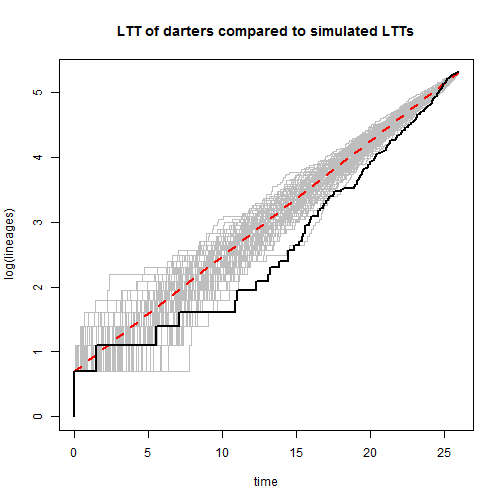``````## Object of class "ltt" containing:
##
## (1) A phylogenetic tree with 201 tips and 200 internal nodes.
##
## (2) Vectors containing the number of lineages (ltt) and branching times (times) on the tree.
##
## (3) A value for Pybus & Harvey's "gamma" statistic of 0.2007, p-value = 0.841.
``````

Alternatively, the function `ltt95` gives a (1-α)% CI for the LTT based on a set of trees. We use it here using a simulated distribution of trees, but this might also be useful if we have, for example, a set of trees from the posterior distribution of a Bayesian analysis:

``````ltt95(trees,log=TRUE)
title(main="LTT of darters compared to simulated LTTs")
``````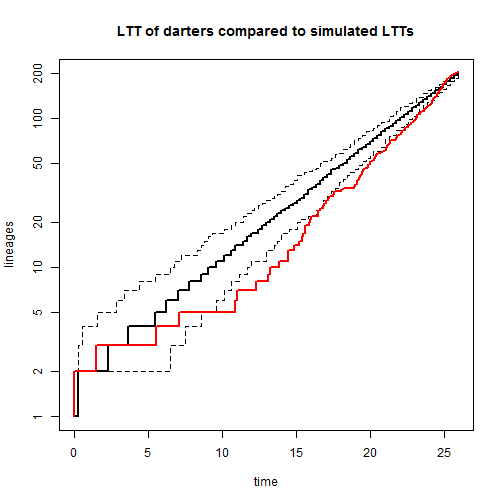``````## Object of class "ltt" containing:
##
## (1) A phylogenetic tree with 201 tips and 200 internal nodes.
##
## (2) Vectors containing the number of lineages (ltt) and branching times (times) on the tree.
##
## (3) A value for Pybus & Harvey's "gamma" statistic of 0.2007, p-value = 0.841.
``````

### The γ-statistic

The γ statistic of Pybus & Harvey (2010) was designed to have a standard normal distribution for trees generated under a pure-birth speciation model. Let's test this with our 100 simulated pure-birth phylogenies:

``````g<-sapply(trees,function(x) ltt(x,plot=FALSE)\$gamma)
hist(g,main=expression(paste("Distribution of ",gamma," from simulation")))
``````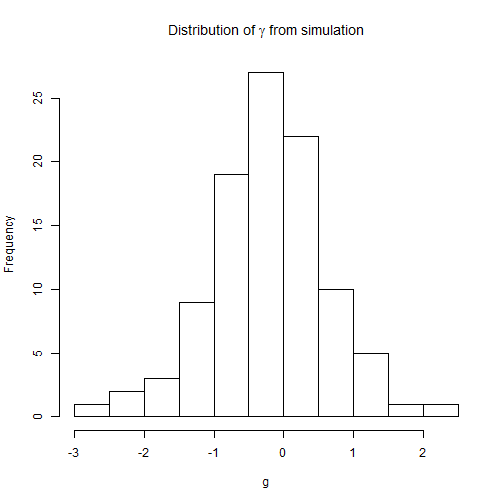``````mean(g)
``````
``````##  -0.1960208
``````
``````var(g)
``````
``````##  0.6981871
``````

We can test hypotheses about γ. This is done automatically with `ltt` or can be done with the function `gammatest` on an object created `ltt`. For example:

``````obj<-ltt(darter.tree,plot=FALSE)
print(obj)
``````
``````## Object of class "ltt" containing:
##
## (1) A phylogenetic tree with 201 tips and 200 internal nodes.
##
## (2) Vectors containing the number of lineages (ltt) and branching times (times) on the tree.
##
## (3) A value for Pybus & Harvey's "gamma" statistic of 0.2007, p-value = 0.841.
``````
``````## or
obj<-ltt(darter.tree,plot=FALSE,gamma=FALSE)
gammatest(obj)
``````
``````## \$gamma
##  0.2006739
##
## \$p
##  0.8409535
``````
``````## multiple trees
obj<-ltt(trees,plot=FALSE)
## type I error
p<-sapply(obj,function(x) x\$p)
mean(p<=0.05) ## should be close to 0.05
``````
``````##  0.04
``````

Finally, let's simulate a tree under a different model of lineage accumulation - the coalescent - and see what the result is. Theoretically, this should result in a significantly positive γ.

``````coal.tree<-rcoal(n=100)
plotTree(coal.tree,ftype="off")
``````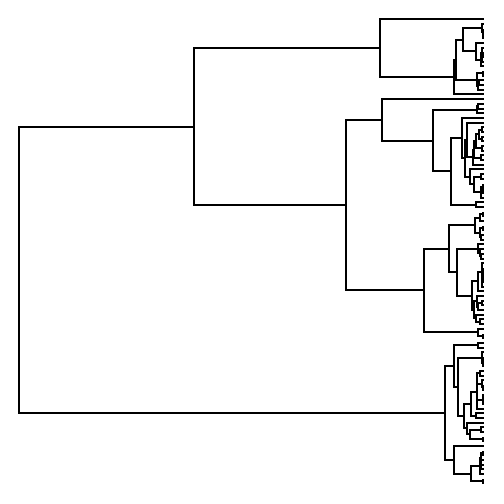``````obj<-ltt(coal.tree,log.lineages=FALSE,log="y")
``````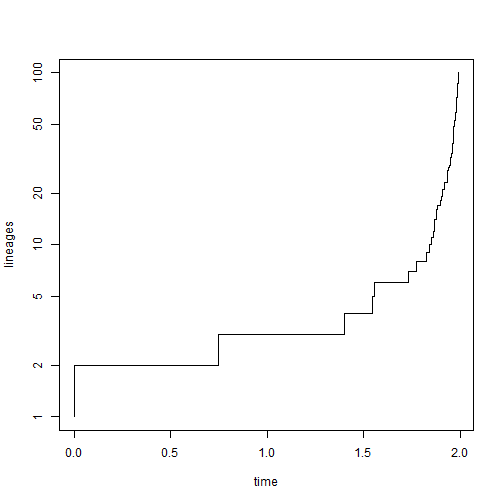``````print(obj)
``````
``````## Object of class "ltt" containing:
##
## (1) A phylogenetic tree with 100 tips and 99 internal nodes.
##
## (2) Vectors containing the number of lineages (ltt) and branching times (times) on the tree.
##
## (3) A value for Pybus & Harvey's "gamma" statistic of 11.2238, p-value = 0.
``````
``````## simulate 200 pure-birth trees for comparison
trees<-pbtree(n=100,nsim=200,scale=max(nodeHeights(coal.tree)))
ltt95(trees,log=TRUE)
title(main="Simulated coalescent tree compared to pure-birth LTTs")
``````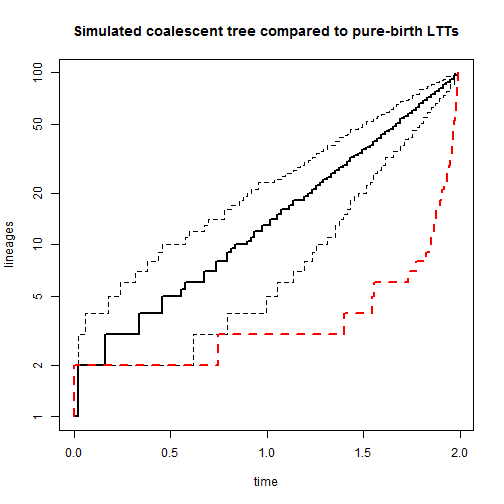``````## Object of class "ltt" containing:
##
## (1) A phylogenetic tree with 100 tips and 99 internal nodes.
##
## (2) Vectors containing the number of lineages (ltt) and branching times (times) on the tree.
##
## (3) A value for Pybus & Harvey's "gamma" statistic of 11.2238, p-value = 0.
``````

Written by Liam J. Revell. Last updated 2 August 2017.